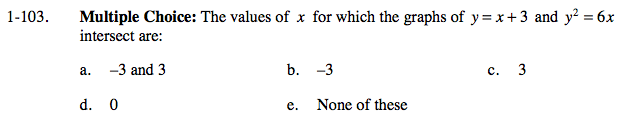### Home > CALC > Chapter 1 > Lesson 1.3.1 > Problem1-103

1-103.Solve the equation y = x + 3 for x.

Does this equation have any solutions?

Substitute this value into the second equation. y² = 6(y − 3)

Set this equation equal to 0. y² − 6y + 18 = 0